# CBSE Class 12 Board Exam Chemistry Paper 2018 2019 2020 With Solutions

Here we are providing CBSE Previous Year Question Papers Class 6 to 12 solved with soutions CBSE Class 12 Board Exam Chemistry Paper 2018 2019 2020 with Solutions Chemistry sample paper class 12, Chemistry previous year question paper class 12, cbse class 12 Chemistry sample paper, cbse class 12 Chemistry sample paper 2020, Chemistry sample paper class 12 2020, cbse sample paper 2020 class 12 Chemistry, class Practice of previous year question papers and sample papers protects each and every student to score bad marks in exams.If any student of CBSE Board continuously practices last year question paper student will easily score high marks in tests. Fortunately earlier year question papers can assist the understudies with scoring great in the tests. Unraveling previous year question paper class 12 Chemistry is significant for understudies who will show up for Class 12 Board tests.

## Class 12 Subject Chemistry Paper Set 1 with Solutions

SECTION A

Read the given passage and answer the questions number 1 to 5 that follow :

The substitution reaction of alkyl halide mainly occurs by SN1 or SN2 mechanism. Whatever mechanism alkyl halides follow for the substitution reaction to occur, the polarity of the carbon halogen bond is responsible for these substitution reactions. The rate of SN1 reactions are governed by the stability of carbocation whereas for SN2 reactions steric factor is the deciding factor. If the starting material is a chiral compound, we may end up with an inverted product or racemic mixture depending upon the type of mechanism followed by alkyl halide. Cleavage of ethers with HI is also governed by steric factor and stability of carbocation, which indicates that in organic chemistry, these two major factors help us in deciding the kind of product formed.

Question 1: (Marks 1)

Predict the stereochemistry of the product formed if an optically active alkyl halide undergoes substitution reaction by SN1 mechanism.

Answer :

Racemic Mixture

Question 2: (Marks 1)

Name the instrument used for measuring the angle by which the plane polarised light is rotated.

Answer :

Polarimeter

Question 3: (Marks 1)

Predict the major product formed when 2-Bromopentane reacts with alcoholic KOH.

Answer :

Pent-2-ene / CH3CH=CHCH2CH3

Question 4: (Marks 1)

Give one use of CHI3 .

Answer :

Antiseptic

Question 5: (Marks 1)

Write the structures of the products formed when anisole is treated with HI.

Answer :

CH3I + C6H5OH

Questions number 6 to 10 are one word answers :

Question 6: (Marks 1)

Identify which liquid will have a higher vapour pressure at 90°C if the boiling points of two liquids A and B are 140°C and 180°C, respectively.

Answer :

A

Question 7: (Marks 1)

Out of zinc and tin, whose coating is better to protect iron objects ?

Answer :

Zn

Question 8: (Marks 1)

Will the rate constant of the reaction depend upon T if the Eact (activation energy) of the reaction is zero ?

Answer :

No

Question 9: (Marks 1)

Give the structure of the monomer of PVC.

Answer :

CH2=CH-Cl

Question 10: (Marks 1)

Which structural unit present in a detergent makes it non-biodegradable ?

Answer :

Branched hydrocarbon part

Questions number 11 to 15 are multiple choice questions :

Question 11: (Marks 1)

Out of the following, the strongest base in aqueous solution is

(A) Methylamine

(B) Dimethylamine

(C) Trimethylamine

(D) Aniline

Answer :

(B) Dimethylamine

Question 12: (Marks 1)

Iodoform test is not given by

(A) Ethanol

(B) Ethanal

(C) Pentan-2-one

(D) Pentan-3-one

Answer :

(D) Pentan-3-one

Question 13: (Marks 1)

Out of the following transition elements, the maximum number of oxidation states are shown by

(A) Sc (Z = 21)

(B) Cr (Z = 24)

(C) Mn (Z = 25)

(D) Fe (Z = 26)

Answer :

(C) Mn (Z = 25)

Question 14: (Marks 1)

Hardening of leather in tanning industry is based on

(A) Electrophoresis

(B) Electro-osmosis

(C) Mutual coagulation

(D) Tyndall effect

Answer :

(C) Mutual coagulation

Question 14: (Marks 1)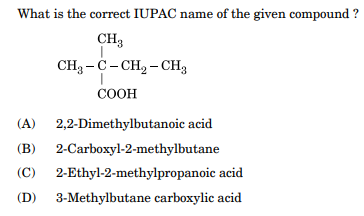Answer :

(A) 2,2-Dimethylbutanoic acid

For questions number 16 to 20, two statements are given  one labelled Assertion (A) and the other labelled Reason (R). Select the correct answer to these questions from the codes (i), (ii), (iii) and (iv) as given below

(i) Both Assertion (A) and Reason (R) are correct statements, and Reason (R) is the correct explanation of the Assertion (A).

(ii) Both Assertion (A) and Reason (R) are correct statements, but Reason (R) is not the correct explanation of the Assertion (A).

(iii) Assertion (A) is correct, but Reason (R) is incorrect statement.

(iv) Assertion (A) is incorrect, but Reason (R) is correct statement.

Question 16: (Marks 1)

Assertion (A) : Au and Ag are extracted by leaching their ores with a dil. solution of NaCN.

Reason (R) : Impurities associated with these ores dissolve in NaCN.

Answer :

(iii) Assertion (A) is correct, but Reason (R) is incorrect statement.

Question 17: (Marks 1)

Assertion (A) : F – F bond in F2 molecule is weak.

Reason (R) : F atom is small in size.

Answer :

(ii) Both Assertion (A) and Reason (R) are correct statements, but Reason (R) is not the correct explanation of the Assertion (A).

Question 18: (Marks 1)

Assertion (A) : Linkage isomerism arises in coordination compounds because of ambidentate ligand.

Reason (R) : Ambidentate ligand like NO2 has two different donor atoms i.e., N and O.

Answer :

(i) Both Assertion (A) and Reason (R) are correct statements, and Reason (R) is the correct explanation of the Assertion (A).

Question 19: (Marks 1)

Assertion (A) : Sucrose is a non-reducing sugar.

Reason (R) : Sucrose has glycosidic linkage.

Answer :

(ii) Both Assertion (A) and Reason (R) are correct statements, but Reason (R) is not the correct explanation of the Assertion (A).

Question 20: (Marks 1)

Assertion (A) : The molecularity of the reaction H2 + Br2 → 2HBr appears to be 2.

Reason (R) : Two molecules of the reactants are involved in the given elementary reaction.

Answer :

(i) Both Assertion (A) and Reason (R) are correct statements, and Reason (R) is the correct explanation of the Assertion (A).

SECTION B

Question 21: (Marks 2)

Define the following terms :

(a) Tranquilizers

(b) Antiseptic

OR

Explain the cleansing action of soaps.

Answer :

(a) The drugs which are used to control stress / anxiety / tension / mild or severe mental diseases

(b) The drugs which are used to kill or to prevent the growth of micro-organism, applied externally on living tissues.

OR

Soap molecules form micelle around the oil droplet or dirt in such a way that hydrophobic part interacts with the oil droplet and hydrophilic part projects out. Micelles can be washed away on rinsing with water. Thus soap helps in emulsification and washing away of oil and fats.

Question 22: (Marks 2)

For a 5% solution of urea (Molar mass = 60 g/mol), calculate the osmotic pressure at 300 K. [R = 0·0821 L atm K–1 mol–1]

OR

Visha took two aqueous solutions — one containing 7·5 g of urea (Molar mass = 60 g/mol) and the other containing 42·75 g of substance Z in 100 g of water, respectively. It was observed that both the solutions froze at the same temperature. Calculate the molar mass of Z.

Answer :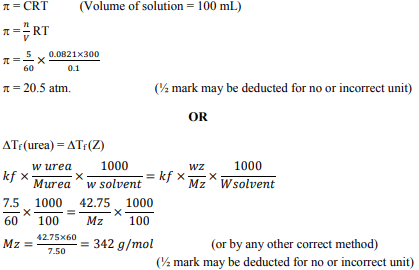Question 23: (Marks 2)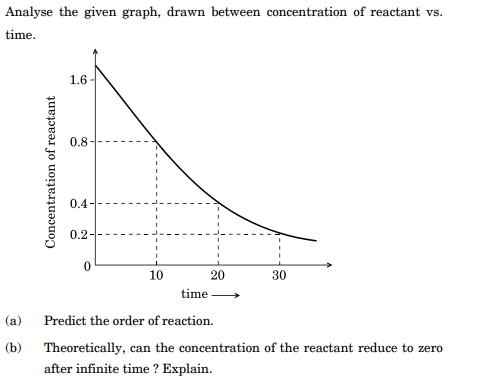Answer :

(a) 1st order

(b) No, due to exponential relation / the curve never touches the x-axis.

Question 24: (Marks 2)

Draw the shape of the following molecules :

(a) XeOF4

(b) BrF3

Answer :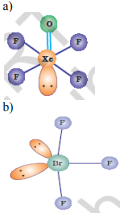Question 25: (Marks 2)

Give the formulae of the following compounds :

(a) Potassium tetrahydroxidozincate (II)

(b) Hexaammineplatinum (IV) chloride

Answer :

(a) K2[Zn(OH)4]

(b) [Pt(NH3)6]Cl4

Question 26: (Marks 2)

What happens when

(a) Propanone is treated with methylmagnesium iodide and then hydrolysed, and

(b) Benzene is treated with CH3COCl in presence of anhydrous AlCl3?

Answer :

a) (CH3)3C-OH / tertiary butyl alcohol is formed

b) C6H5COCH3 / acetophenone is formed (or correct chemical equation)

Question 27: (Marks 2)

Write the names and structures of monomers in the following polymers :

(a) Bakelite

(b) Neoprene

Answer :

a) C6H5OH + HCHO , Phenol + formaldehyde

b) CH2= C(Cl) – CH=CH2, Chloroprene

SECTION C

Question 28: (Marks 3)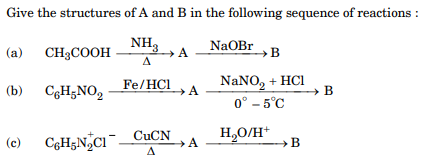OR

(a) How will you distinguish between the following pairs of compounds :

(i) Aniline and Ethanamine

(ii) Aniline and N-methylaniline

(b) Arrange the following compounds in decreasing order of their boiling points :

Butanol, Butanamine, Butane

Answer :

(a) (A) → CH3CONH2 (B) → CH3NH2

(b) (A) → C6H5NH2 (B) → C6H5N2Cl

(c) (A) → C6H5CN (B) → C6H5COOH

OR

a) (i) Add Ice cold (NaNO2 + HCl) followed by phenol or β-Naphthol to both the compounds.

Aniline forms orange red dye while ethylamine doesn’t.

ii) Add CHCl3 and KOH (alc.) to both the compounds.

Aniline gives foul smelling isocyanide while N-Methylaniline doesn’t.

(or any other suitable chemical test)

b) Butanol > Butanmine > Butane

Question 29: (Marks 3)

Give the plausible explanation for the following :

(a) Glucose doesn’t give 2,4-DNP test.

(b) The two strands in DNA are not identical but are complementary.

(c) Starch and cellulose both contain glucose unit as monomer, yet they are structurally different.

Answer :

(a) Because the – CHO group in glucose is involved in hemiacetal formation and thus is not free / due to cyclic structure of glucose -CHO group is not free.

(b) Because the hydrogen bonds are formed between specific pairs of bases.

(c) Starch is a polymer of α - glucose while cellulose is a polymer of β - glucose.

Question 30: (Marks 3)

Account for the following :

(a) Sulphurous acid is a reducing agent.

(b) Fluorine forms only one oxoacid.

(c) Boiling point of noble gases increases from He to Rn.

OR

Complete the following chemical reactions :

(a) MnO2 + 4 HCl →

(b) XeF6 + KF →

(c) I – (aq) + H+ (aq) + O2 (g) →

Answer :

(a) Because sulphur readily gets oxidized itself to more stable +6 state.

(b) Because of absence of d-orbital in Fluorine.

(c) Because size increases from Helium to Radon. / dispersion or van der Waal forces increase from Helium to Radon.

OR

(a) MnO2+ 4HCl → MnCl2 + Cl2 + 2H2O

(b) XeF6 + KF → K + [XeF7]-

(c) 4I-(aq.) + 4H+(aq.) + O2(g) → 2I2(s) + 2H2O(l)

Question 31: (Marks 3)

Explain the role of the following :

(a) NaCN in the separation of ZnS and PbS.

(b) SiO2 in the metallurgy of Cu containing Fe as impurity.

(c) Iodine in the refining of Ti.

Answer :

(a) NaCN act as a depressant.

(b) SiO2 act as a flux. / used to remove FeO as slag

(c) I2 is used to convert Ti into volatile compound (TiI4).

Question 32: (Marks 3)

Give three points of difference between physisorption and chemisorption.

Answer :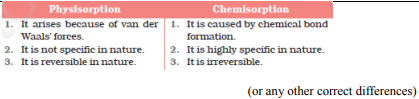Question 33: (Marks 3)

How will the rate of the reaction be affected when

(a) Surface area of the reactant is reduced,

(b) Catalyst is added in a reversible reaction, and

(c) Temperature of the reaction is increased ?

Answer :

(a) Decreases.

(b) Increases

(c) Increases

Question 34: (Marks 3)

Calculate the mass of ascorbic acid (Molar mass = 176 g mol–1) to be dissolved in 75 g of acetic acid, to lower its freezing point by 1·5°C. (Kf = 3·9 K kg mol–1)

Answer :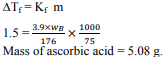SECTION D

Question 35: (Marks 5)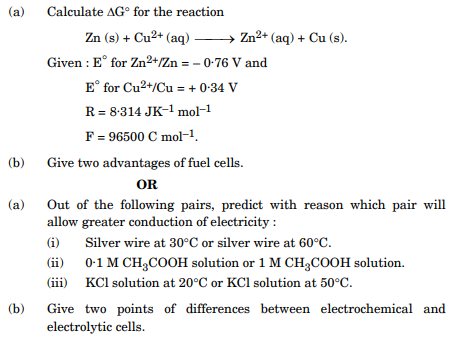Answer :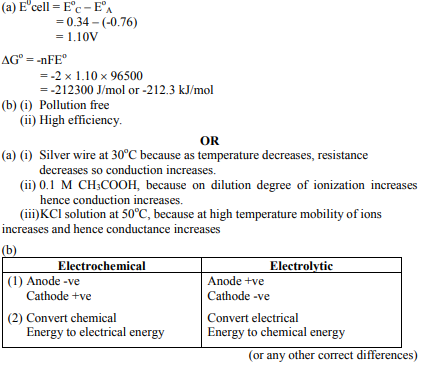Question 36: (Marks 5)

(a) Account for the following :

(i) Copper (I) compounds are white whereas Copper (II) compounds are coloured.

(ii) Chromates change their colour when kept in an acidic solution.

(iii) Zn, Cd, Hg are considered as d-block elements but not as transition elements.

(b) Calculate the spin-only moment of Co2+ (Z = 27) by writing the electronic configuration of Co and Co2+.

OR

(a) Give three points of difference between lanthanoids and actinoids.

(b) Give reason and select one atom/ion which will exhibit asked property :

(i) Sc3+ or Cr3+ (Exhibit diamagnetic behaviour)

(ii) Cr or Cu (High melting and boiling point)

Answer :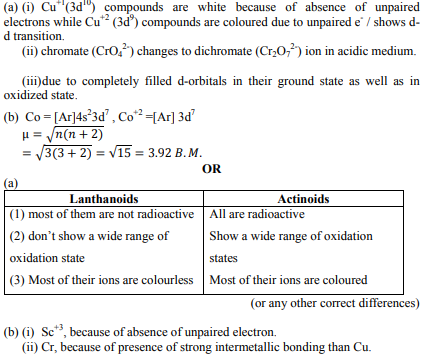Question 37: (Marks 5)

(a) Out of t-butyl alcohol and n-butanol, which one will undergo acid catalyzed dehydration faster and why ?

(b) Carry out the following conversions :

(i) Phenol to Salicylaldehyde

(ii) t-butylchloride to t-butyl ethyl ether

(iii) Propene to Propanol

OR

(a) Give the mechanism for the formation of ethanol from ethene.

(b) Predict the reagent for carrying out the following conversions :

(i) Phenol to benzoquinone

(ii) Anisole to p-bromoanisole

(iii) Phenol to 2,4,6-tribromophenol

Answer :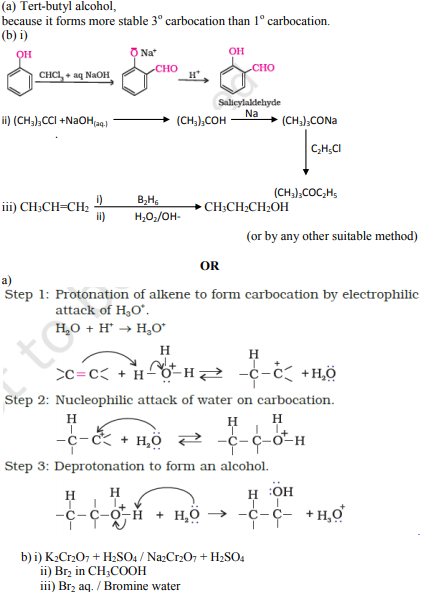☞ Click here for privious year Question papers

## Chemistry sample paper class 12, Chemistry previous year question paper class 12, cbse class 12 Chemistry sample paper, cbse class 12 Chemistry sample paper 2020, Chemistry sample paper class 12 2020, cbse sample paper 2020 class 12 Chemistry, class 12 Chemistry sample paper 2020, class 12 important questions Chemistry, cbse class 12 board exam Chemistry paper, Chemistry previous year question papers class 12 with solutions, Chemistry sample paper class 12 2019, cbse class 12 Chemistry question paper 2017 solved pdf, cbse class 12 Chemistry question paper 2018, class 12 Chemistry paper 2019, Chemistry question paper for class 12, cbse class 12 Chemistry paper 2019

### NCERT Books Free Pdf Download for Class 5, 6, 7, 8, 9, 10 , 11, 12 Hindi and English Medium

 Mathematics Biology Psychology Chemistry English Economics Sociology Hindi Business Studies Geography Science Political Science Statistics Physics Accountancy

## Please Share this webpage on facebook, whatsapp, linkdin and twitter.

Copyright @ ncerthelp.com A free educational website for CBSE, ICSE and UP board.